Courses

# Calculation Of Number Of Particles In A Unit Cell (Z) - Solid State Chemistry Notes | EduRev

## Chemistry : Calculation Of Number Of Particles In A Unit Cell (Z) - Solid State Chemistry Notes | EduRev

The document Calculation Of Number Of Particles In A Unit Cell (Z) - Solid State Chemistry Notes | EduRev is a part of the Chemistry Course Physical Chemistry.
All you need of Chemistry at this link: Chemistry

Calculation of number of particles in a unit cell (Z)

In a crystal atoms located at the corner and face centre of a unit cell are shared by other cells and opnly a portion of such an atom actually lies within a given unit cell.

(i) A point that lies at the corner of a unit cell is share among eight unit cells and therefore, only one eighth of each such point lies within the given unit cell.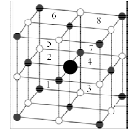(ii) A point along an edge is shared by four unit cells and only one-fourth of it lies within any one cell.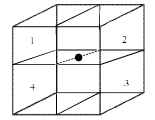(iii) A face-centred point is shared by two unit cells and only one half of it is present in a given unit cell.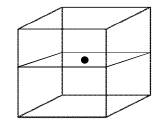(iv) A body-centred point lies entirely within the unit cell and contributes one complete point to the cell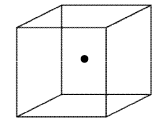Type of Lattice point Contribution to one unit cell Corner 1/8 Edge ¼ Face-center ½ Body centre 1

Calculate the number of particles in a unit cell

 Type of unit cell Lattice points at corner Lattice points at face-centered Lattice points at body centered Z = no. of lattice points per unit cell SSC 8 0 0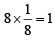BCC 8 0 1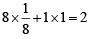FCC 8 6 0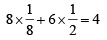Relation between edge length of unit cell and radius of atoms

(i) Simple cubic or Primitive

(a) edge length of unit cell a = 2r (b) atomic radius r = a/2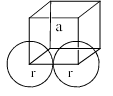(ii) Body centre cubic

(a) edge length of unit cell =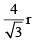(b) atomic radius, r =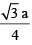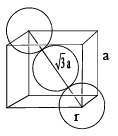(iii) Face centre cubic/cubic close packed

(a) edge length of unit cell a =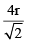(b) atomic radius r =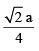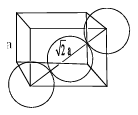Illustration: A solid has a cubic structure in which X atoms are located at the corners of the cube, Y atoms are at the cube centres and O atoms are at the edge centres. What is the formula of the compound:

Solution: Atoms of X are present at all the eight corners of the cube. Therefore, each atom of X at the corner makes 1/8 contribution towards the unit cell.
Number of atoms of X per unit cell =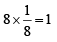Y atom is present at the body centre, thus contribution of Y towards unit cell = 1 × 1 = 1
O atom is present at each of the edge centre (number of edges of cube = 12)
And each O atom present at edge centre will make ¼ contribution towards the unit cell.

The number of O atoms per unit cell =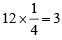The formula of the compound is, therefore XYO3

Fraction of volume occupied by atoms in a cube

Packing Fraction = Volume of atoms in the cube/Total volume of cube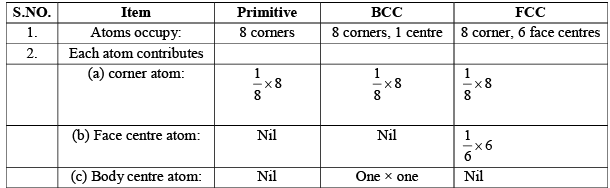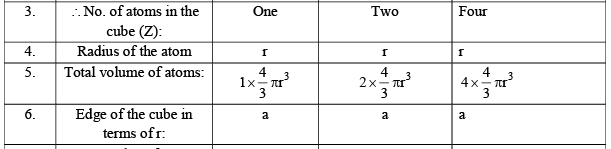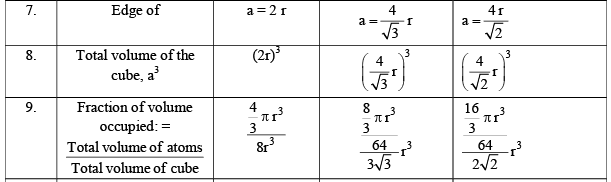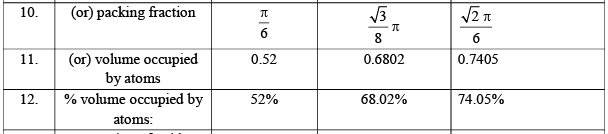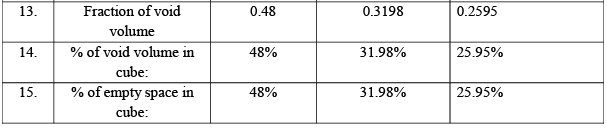Density of unit cell

Density of unit cell (and hence density of a crystal)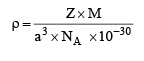Where a is edge of unit cell in pm
NA = Avogadro number (6.02 × 1023)
M = Atomic mass of element or formula mass of the compound
Z = No. Of toms present per unit cell or formula units e.g. for fcc, Z = 4, for bcc, Z = 2, for simple cubic, Z = 1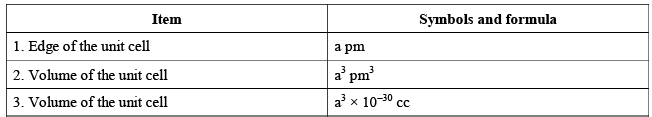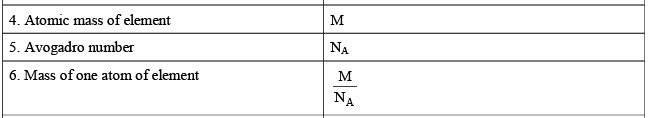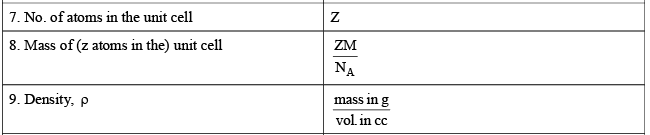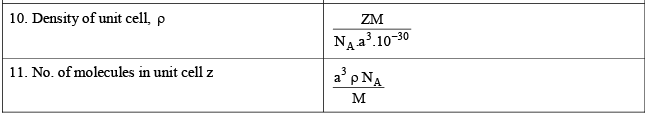Illustration: Gold has a close-packed structure which can be viewed as-spheres occupying 0.74 of the total volume. If the density of gold is 19.3 g/cc, calculate the apparent radius of a gold ion in the solid

Solution: Gold has s close-packed structure with a packing fraction value of 0.74. This shows that it has a face-centred cubic cell. The number of ions in a face-centred unit cell is 4.
Now density =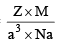Or 19.3 =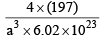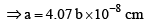In a face-centred cubic cell.
Radius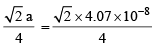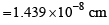Offer running on EduRev: Apply code STAYHOME200 to get INR 200 off on our premium plan EduRev Infinity!

## Physical Chemistry

66 videos|94 docs|32 tests

,

,

,

,

,

,

,

,

,

,

,

,

,

,

,

,

,

,

,

,

,

;